# Adding waves (of the same frequency) together

• When two sinusoidal waves with identical frequencies and wavelengths interfere, the result is another wave with the same frequency and wavelength, but a maximum amplitude which depends on the phase difference between the input waves.
• Constructive interference occurs when the phase difference between two waves is zero, or some multiple of pi radians
• Destructive interference occurs when the phase difference between two waves is some multiple of pi/2 radians
• Adding two sound waves which are out of phase may yield silence!
•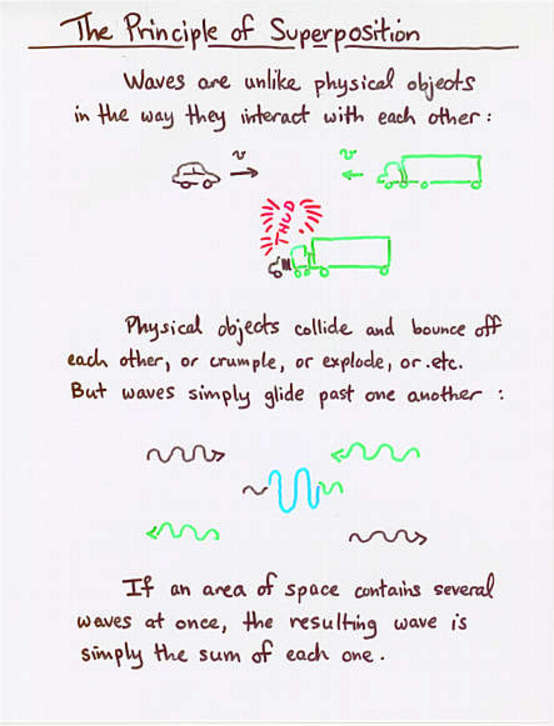Viewgraph 0

•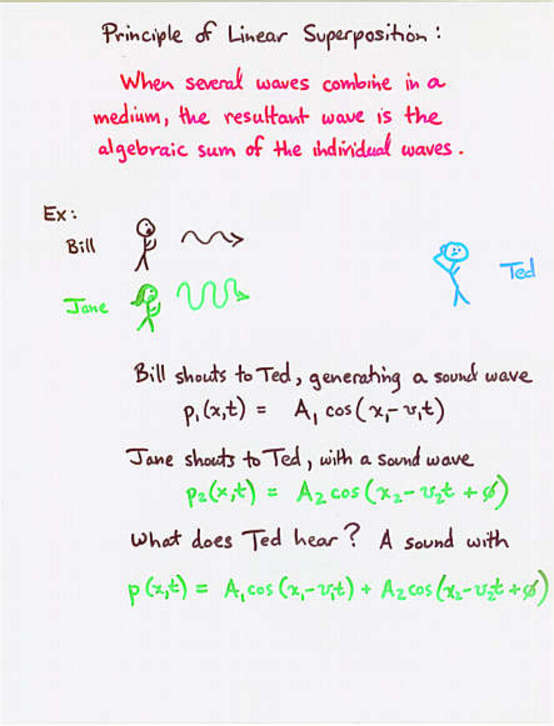Viewgraph 1

•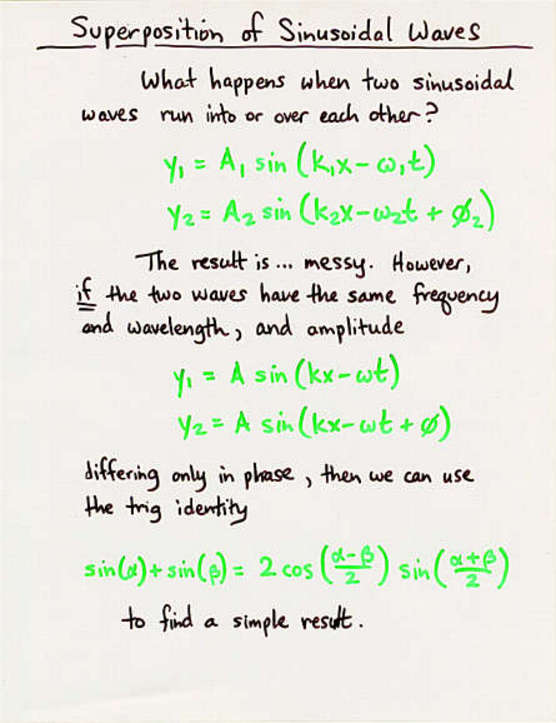Viewgraph 2

•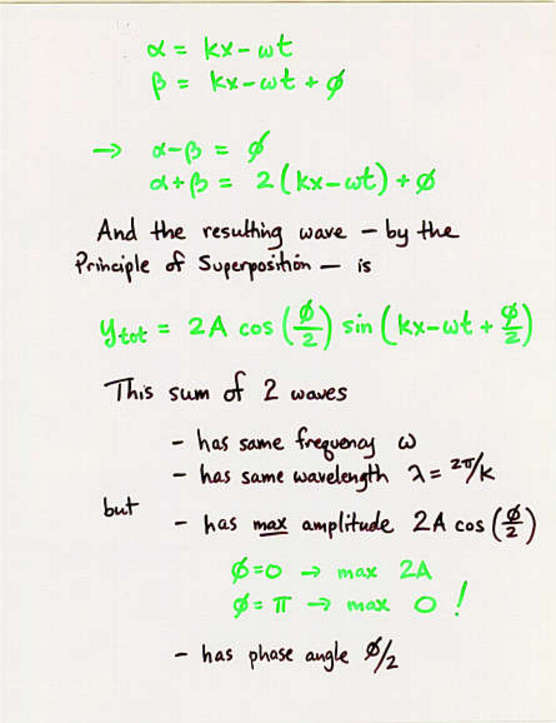Viewgraph 3

•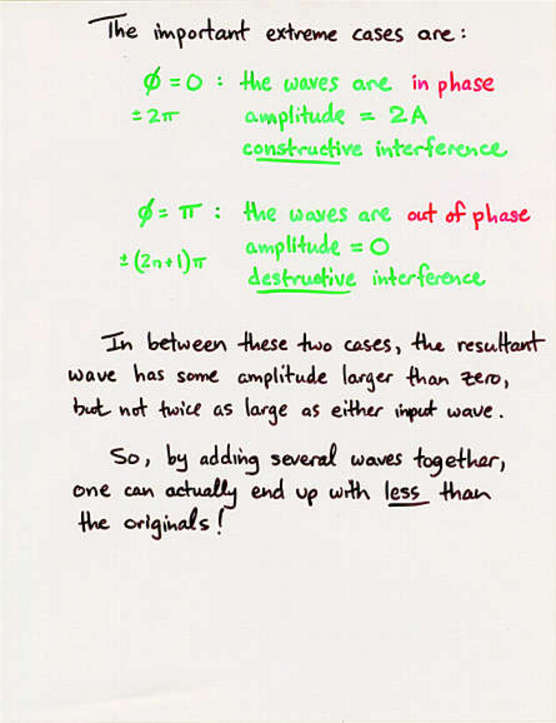Viewgraph 4

```

Q:  Two travelling waves meet:

y1  =  (5 cm) * sin ( 3 rad/m * x  -  0.1 rad/s * t )

y2  =  (5 cm) * sin ( 3 rad/m * x  -  0.1 rad/s * t  +  1 rad )

Is the amplitude of the combined wave

a)  bigger than either original wave

b)  smaller than either original wave

c)  the same as either original wave

What is the SPEED of this combined wave?

```
•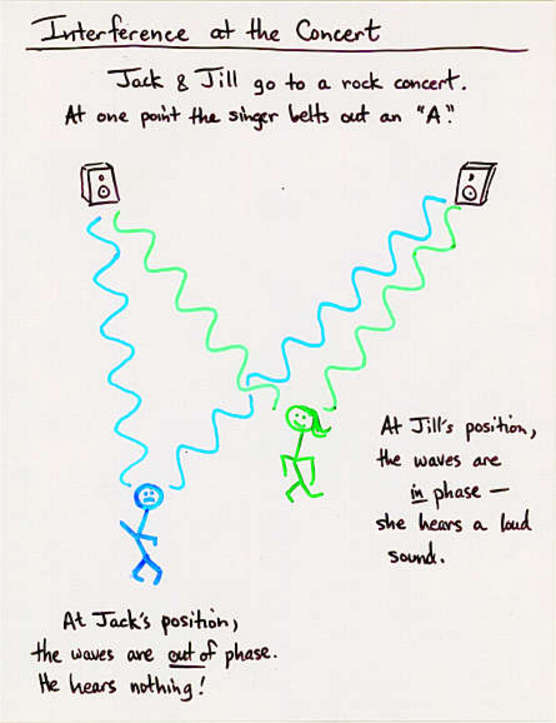Viewgraph 5

•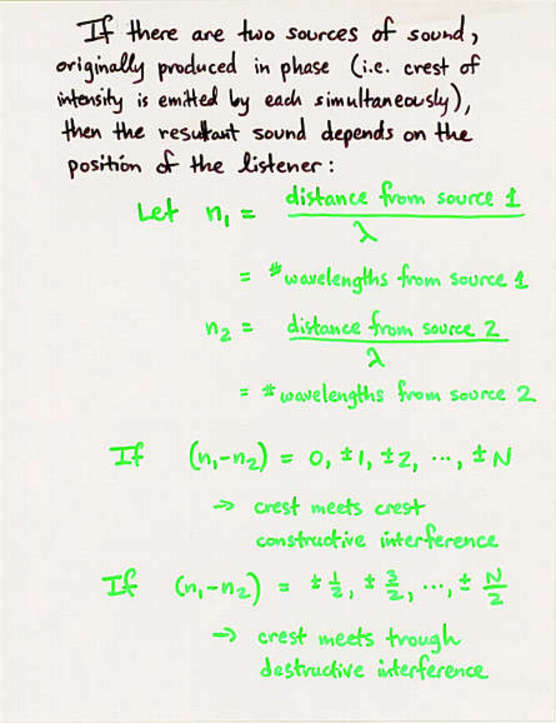Viewgraph 6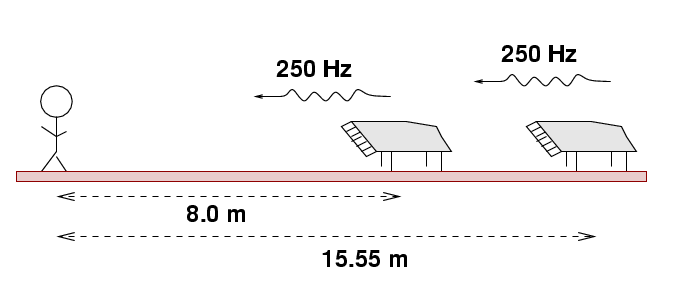Joe goes to the concert hall and hears two pianos played at once. Each piano strikes an identical 250 Hz note.

```

Q:  Does Joe hear constructive interference,
destructive interference, or something
in between?

```